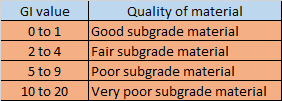## Translate

### How to calculate Static Indeterminacy of a structure

• A statically determinate structure is such structure for which conditions of static equilibrium equations are sufficient to analyze the structure.  While for the indeterminate structures,  static equilibrium equations are not sufficient to analyze the structure.
• This means that in indeterminate structure,  the number of unknown forces in members or the support reactions are in excess of number of static equilibrium equation available.
• So,  the static Indeterminacy can be defined as the difference between total number of unknown forces and total number of equilibrium equation available.
Ds = (total unknown) - (available equation)

## Static Indeterminacy for beams

• The basic rule to calculate the static Indeterminacy for any structures is to find the difference between total number of unknown and total number of equilibrium equation available.
• To,  determine static Indeterminacy for beams,  the simple procedure is that one should try to make given beam as a cantilever beam by adding constraints and removing all other support reactions.
Ds= ( support removed - constraint added)
• Note:-  Internal Indeterminacy of a beam is always zero, i.e, beam is always internally determinate and for beam, total Indeterminacy is always equal to external Indeterminacy.

## Static Indeterminacy for truss

• The basic rule to calculate the static Indeterminacy for any structures is to find the difference between total number of unknown and total number of equilibrium equation available.
• In truss, member only carries axial force and no other internal reactions. So,  each member of a truss will give one unknown.  And the reactions developed at support will also constitute for the total number of unknown.
• Now, at each of the joint present in the structure, the force equilibrium equation will be available.  So,  the number of equations will be ( 2 each for plane truss)  and ( 3 each for space truss).
• Ds = (m+r) - 2j 👉  plane truss
• Ds = (m+r) -3j 👉   space truss
• where m = number of member
• n = number of reactions
• j = number of joints
•  Note:- External Indeterminac
•  (r-3) for plane truss
• (r-6) for space truss

• Internal Indeterminacy
• [m - (2j-3)] for plane truss
• [m - (3j-6)] for space truss

## Static indeterminacy for Rigid Frame

•  The basic rule to calculate the static Indeterminacy for any structures is to find the difference between total number of unknown and total number of equilibrium equation available.
• In rigid plane frame,  each member carries 3 forces and in case of space frame,  each member carries 6 forces. And the reactions developed also constitute for the unknowns.
• Now,  at each of the joint present in the structure,  the equilibrium equation will be available. So,  the number of equations will be (3 each for plane frame)  and ( 6 each for space frame).
• Now, for m' member meeting at a hinge location, there will be extra {(m'-1) independent equation for plane frame} and {3(m'-1) independent equation for space frame}.
• Ds = 3m + r - 3j - Σ(m'-1)   👉 plane frame
• Ds = 6m + r - 6j - Σ3(m'-1) 👉 space frame
•  where, m = number of member
• r  = number of reaction
• j = number of joints
• m' = number of members meeting at a hinge